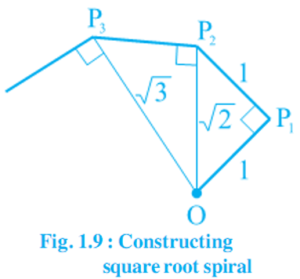chillimath.com

## Ncert Solutions for Class 9

#### Exercise 1.2

1. State whether the following statements are true or false. Justify your answers.

(i) Every irrational number is a real number.

(ii) Every point on the number line is of the form $$\sqrt{m}$$, where m is a natural number.

(iii) Every real number is an irrational number.

2. Are the square roots of all positive integers irrational? If not, give an example of the square root of a number that is a rational number.

3. Show how $$\sqrt5$$ can be represented on the number line.

4. Classroom activity (Constructing the ‘square root spiral’) :  Take a large sheet of paper and construct the square root spiral in the following fashion. Start with a point O and draw a line segment OP1 of unit length. Draw a line segment P1P2 perpendicular to OP1 of unit length (see Fig. 1.9). Now draw a line segment P2P3 perpendicular to OP2. Then draw a line segment P3P4 perpendicular to OP3. Continuing in this manner, you can get the line segment Pn-1Pn by drawing a line segment of unit length     perpendicular to OPn-1. In this manner, you will have created the points P2, P3,…., Pn, … ., and joined them to create a beautiful spiral depicting $$\sqrt 2$$, $$\sqrt 3$$, $$\sqrt 4$$, …